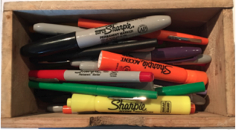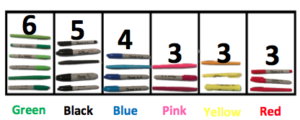How can the concepts found in the Measurement and Data domain be used as an application of other domains?

One of the critical areas in Kindergarten is representing and comparing whole numbers, initially with sets of objects. In the Classifying and sorting units there are ample opportunities to make connections to previously learned content.

For example, as students are sorting every day objects, you can reinforce, MAFS.K.CC.2.5 Count to answer “how many?” questions about as many as 20 things arranged in a line, a rectangular array, or a circle, or as many as 10 things in a scattered configuration; given a number from 1–20, count out that many objects.Students can practice the skills found in the standard, MAFS.K.CC.1.3, Read and write numerals from 0 to 20. Represent a number of objects with a written numeral 0–20 (with 0 representing a count of no objects), by representing the number in each set.Students can also make connections to MAFS.K.CC.3.6, Identify whether the number of objects in one group is greater than, less than, or equal to the number of objects in another group, e.g., by using matching and counting strategies.It is important to help students make connections in math. What other questions could you ask to make connections to previously learned content?